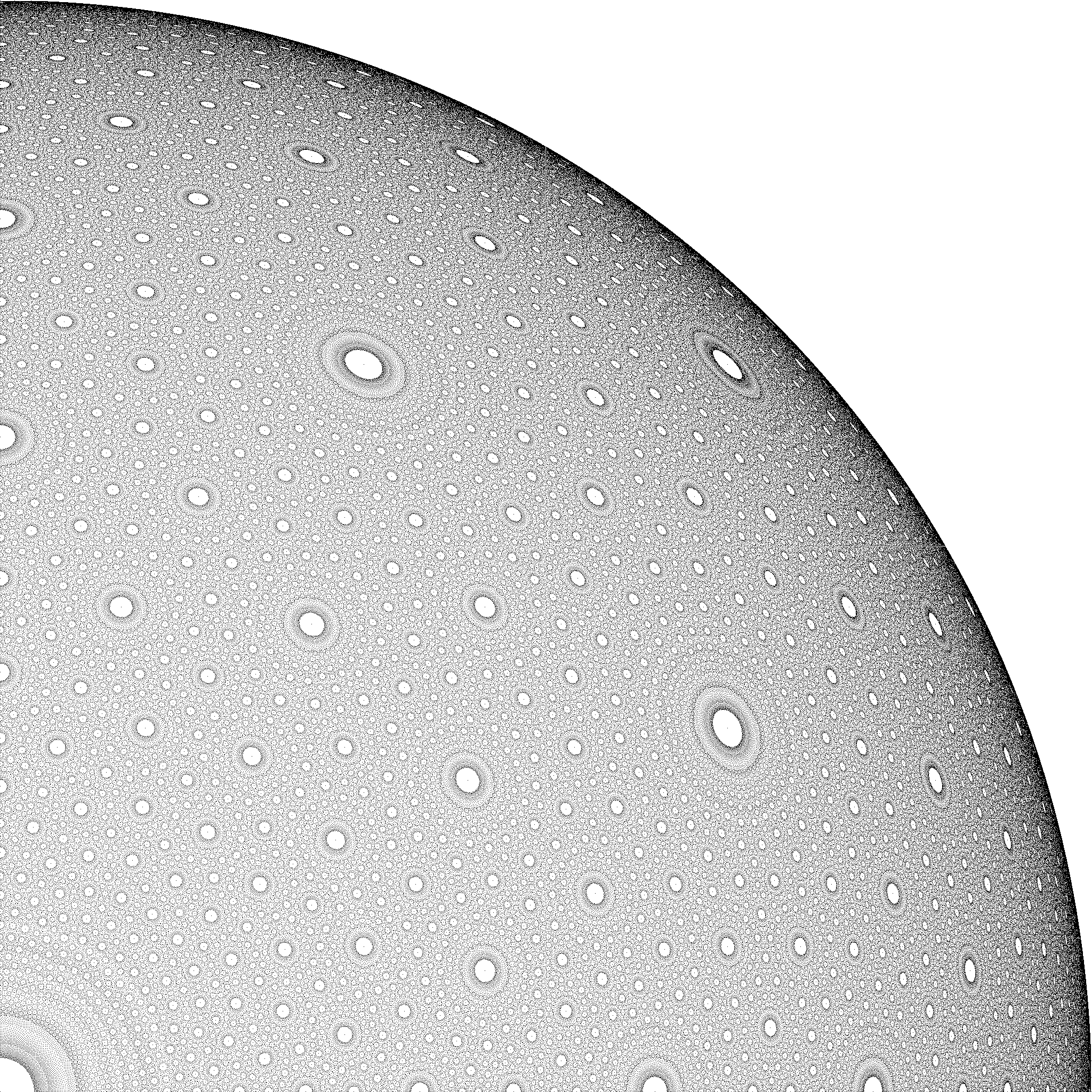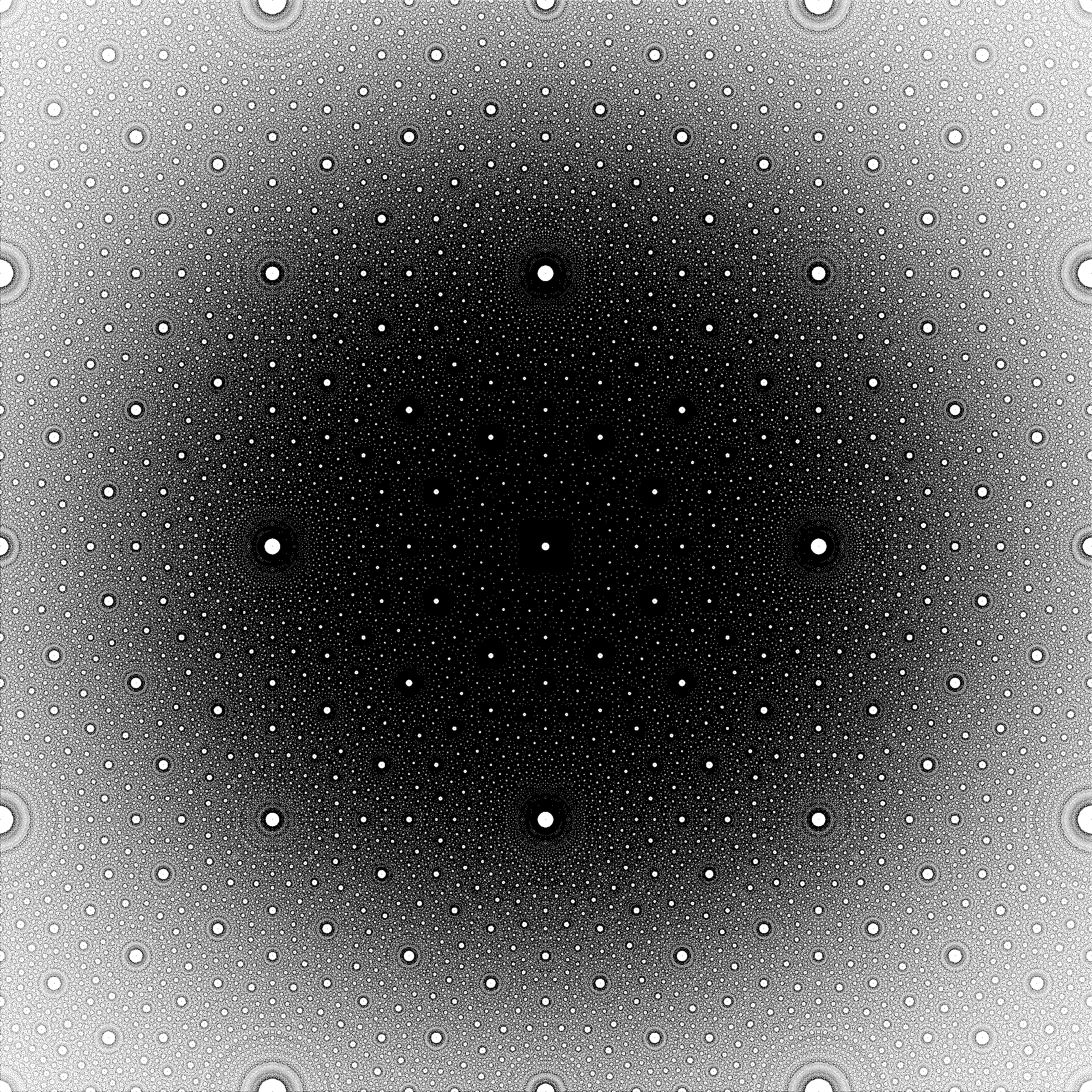# Rational points on a sphere in $\mathbb{R}^d$

Call a point of $\mathbb{R}^d$ rational if all its $d$ coordinates are rational numbers.

Q1. Are the rational points dense on the unit sphere $S :\; x_1^2 +\cdots+ x_d^2 = 1$, i.e. does $S$ contain a dense set of rational points?

This is certainly true for $d=2$, rational points on the unit circle.

Q2. If (as I suspect) the answer to Q1 is Yes, is there a sense in which the rational coordinates are becoming arithmetically more complicated with larger $d$, say in terms of their height?

If $x= a/b$ is a rational number in lowest terms (i.e. gcd$(a,b)=1$), then the height of $x$ is $\max \lbrace |a|,|b| \rbrace$.

This is far from my expertise. No doubt this is known, in which case a pointer would suffice. Thanks!

(Added, 22Mar13). I just found this reference.

Klee, Victor, and Stan Wagon. Old and new unsolved problems in plane geometry and number theory. No. 11. Mathematical Association of America, 1996. p.135.• Stereographic projection makes rational points on the sphere correspond to rational points in $\mathbb R^{d-1}$. – Tom Goodwillie Mar 22 '13 at 1:39
• Yes. And the formula of the stereographic projection is not more complicated in high dimension, so this also answers to question 2. – Jérémy Blanc Mar 22 '13 at 2:14
• The inverse of stereographic projection does map rational points to rational points. – Tom Goodwillie Mar 22 '13 at 11:52
• I don't understand why Peter Michor's comment got two upvotes. If a quadric hypersurface $X \subset \mathbf{A}^{n+1}$ defined over $\mathbf{Q}$ has a point $P \in X(\mathbf{Q})$, then projecting away from $P$ gives a birational map $X \stackrel{\sim}{\dashrightarrow} \mathbf{A}^n$ that is defined over $\mathbf{Q}$. Restricting this birational map gives an isomorphism between open subsets of $X$ and $\mathbf{A}^n$ that is defined over $\mathbf{Q}$. In particular, the rational point son $X$ are dense in the real locus of $X$ iff the same holds for $\mathbf{A}^n$, which is trivially the case. – RP_ Mar 22 '13 at 12:06
• Joseph: One more thing. A sphere contains dense set of rational points if and only if it contains a finite set of rational points whose affine span is the entire affine space. Proving this is an exercise in using rational Moebius transformations, generalizing the argument with stereographic projection which is a restriction of such a Moebius transformation. I guess, Klee and Wagon did not know about this group (which is PO(n,1; Q)). – Misha Mar 23 '13 at 12:04

## 5 Answers

The question itself has already been answered. Let me just add that in case $d = 3$, one can obtain a nice picture by marking all rational points with height less than some upper bound, and projecting this to one of the coordinate planes. The following picture shows such projection of one octant of the sphere (bound on height: 2048):This picture in resolution 2048 x 2048 pixels can be found at https://stefan-kohl.github.io/images/ratpoints2048.png.

Larger versions of this picture are available as well:

Projecting the rational points on the sphere in a Riemann-sphere-like way to the plane yields a picture like this:The point where the sphere touches the plane is in the middle of the picture. One feature of the picture is a grid of white circles with mesh size 2, i.e. the diameter of the sphere. The higher density of points around the middle of the picture arises from the projection.

Larger versions of this picture are available as well:

• That certainly looks interesting; I can't explain the patterns off the top of my head. Can you make an animation where you vary the height bound? I'd be interested to see it if you can. – RP_ Mar 23 '13 at 17:35
• That would be some work. -- Though what you would see is roughly the following: as the height bound increases, the white circles around the points with small height get smaller and smaller, and the points on their boundaries get denser and denser. – Stefan Kohl Mar 23 '13 at 18:11
• The picture is essentially the same for the (projective) plane (via stereographic projection). The reason for the white disks is that points of small height `repel' each other so that nearby you only find points of comparably large height. – Michael Stoll Mar 23 '13 at 19:05
• @Stefan: This is an entirely non-substantive comment, but I cannot but view your incredibly fascinating image as analogous to meteors impacting the surface of a planet, like Jupiter. – Joseph O'Rourke Mar 23 '13 at 21:43
• Can you expand a bit on how you created this picture? How were the individual points obtained? The shading suggests, that you actually created points on a cube and projected them - which led to an increased density where the corners of the cube had been. If you did not create this plot like that it would be very interesting to investigate the origin of the increased densities. – example Aug 17 '14 at 18:03

Coincidentally, I learnt about the following paper (from 2008) by Erich Schmutz in a talk by Amos Nevo this morning which, I believe, answers both of your questions: Rational Points on the Unit Sphere.

In particular, he proves the following bound on the height of a rational point $r = (r_1, \ldots, r_d)$ on the unit sphere which is $\epsilon$-close to $x = (x_1, \ldots, x_d)$ for the sup-norm: $$H(r) \le \left( \frac {\sqrt{32} m} \epsilon \right)^{2 m},$$ where $m = \lceil \log_2 d \rceil$.

• @Daniel: Thanks! We posted links to the same paper at the same time. :-) – Joseph O'Rourke Mar 22 '13 at 19:46

With regards to Q1 you can get a finer notion of density by counting the number $N(B)$, say, of rational points with height at most $B$, and consider how this quantity behaves as $B\rightarrow \infty$. I would think of this problem projectively. In this case rational points would be $[x_1,\dots,x_n,y]$, with $x_1,\dots,x_n,y$ integers such that $\gcd(x_1,\dots,x_n,y)=1$, for which $$x_1^2+\cdots +x_d^2=y^2.$$ One could then define the height of a point to be $\max(|x_i|,|y|)$. Using the Hardy-Littlewood circle method, for example, one can prove (for $d\geq 3$) that $$N(B)\sim B^{d-1}\sigma_\infty\prod_p\sigma_p,$$ as $B\rightarrow \infty$, where $\sigma_v$ is the density of points on the quadric in the completion $\mathbb{Q}_v$.

We (with Keith Merrill) recently wrote a paper further quantifying the density of rational points on $S^n$, see http://arxiv.org/abs/1301.0989. Hope it helps!

• A belated welcome to MO, Dmitri. – Lee Mosher Apr 9 '13 at 23:06

Maybe the following paper and references therein help:

MR1975458 (2004h:11031) Reviewed Margulis, Gregory(1-YALE) Diophantine approximation, lattices and flows on homogeneous spaces. A panorama of number theory or the view from Baker's garden (Zürich, 1999), 280–310, Cambridge Univ. Press, Cambridge, 2002.

• @Peter: A lacuna in our libraries here, so Interlibrary loan to the rescue... – Joseph O'Rourke Mar 22 '13 at 11:30